###### Understanding Attention Mechanism in Sequence 2 Sequence Machine Translation
2021-08-22###### SumTree data structure for Prioritized Experience Replay (PER) explained with Python Code
2021-09-09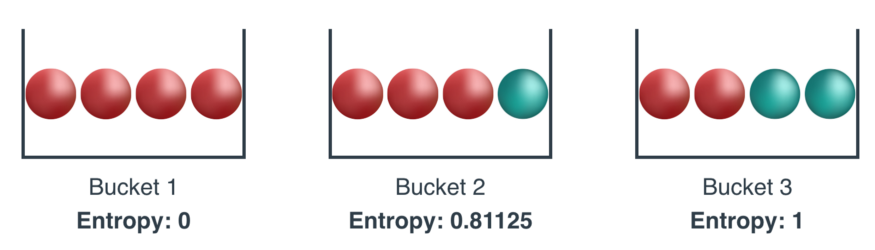Suppose you are talking with three patients in the waiting room of a doctor’s office. All three of them have just completed a medical test which, after some processing, yields one of two possible results: the disease is either present or absent. Let’s assume we are dealing with curious and data-oriented individuals. They’ve researched the probabilities for their specific risk profiles in advance and are now eager to find out the result.

Patient A knows that, statistically, there is a 95% chance that he has the disease in question. For Patient B, the probability of being diagnosed with an illness is 30%. Patient C, in contrast, faces a 50/50 probability.

I would like to focus on a simple question. All other things being equal, which of the three patients is confronted with the greatest degree of uncertainty?

I think the answer is clear: patient C. Not only is he experiencing “a lot of uncertainty”. What he is going through is the greatest degree of uncertainty possible under the circumstances: a dramatic medical version of a flip with a fair coin.

Compare this with patient A. Sure, the overall situation looks quite grim, but at least this patient is experiencing little uncertainty with regard to his medical prospects.

Intuitively speaking, what can we say about patient B? Perhaps that her situation falls “somewhere in the middle”?

This is where entropy comes in. Describing a situation as “somewhere in the middle” might be good enough for waiting room talk, but it’s certainly too coarse a description for machine learning purposes.

## Measuring uncertainty

Entropy allows us to make precise statements and perform computations with regard to one of life’s most pressing issues: not knowing how things will turn out.

Entropy, in other words, is a measure of uncertainty.

(It is also a measure of information, but, personally, I prefer the uncertainty interpretation. It might just be me, but things seemed a lot clearer when I no longer attempted to impose my preconceived notion of information on the equations.)

In a way, saying that entropy is “a measure of uncertainty” is an understatement. Given certain assumptions (and foreshadowing an important result mentioned below), entropy is the measure of uncertainty.

By the way, when I use the term entropy, I’m referring to Shannon entropy. There are quite a few other entropies, but I think it’s safe to assume that Shannon entropy is the one that is used most frequently in natural language processing and machine learning.

So without further ado, here it is, the entropy formula for an event X with n possible outcomes and probabilities p_1, …, p_n:

# Basic properties

If you are anything like me when I first looked at this formula, you might be asking yourself questions such as: Why the logarithm? Why is this a good measure of uncertainty at all? And, of course, why the letter H? (Apparently, the use of the English letter H evolved from the Greek capital letter Eta, although the history appears to be quite complicated.)

One thing I’ve learned over time is that a good starting point — here and in many other cases — is to ask two questions: (1) Which desirable properties does the mathematical construct I’m trying to understand have? And (2) Are they any competing constructs that have all of these desirable properties?

In short, the answers for Shannon entropy as a measure of uncertainty are (1) many and (2) no.

Let’s proceed with a wish list.

## Basic property 1: Uniform distributions have maximum uncertainty

If your goal is to minimize uncertainty, stay away from uniform probability distributions.

Quick reminder: A probability distribution is a function that assigns a probability to every possible outcome such that the probabilities add up to 1. A distribution is uniform when all of the outcomes have the same probability. For example, fair coins (50% tails, 50% tails) and fair dice (1/6 probability for each of the six faces) follow uniform distributions.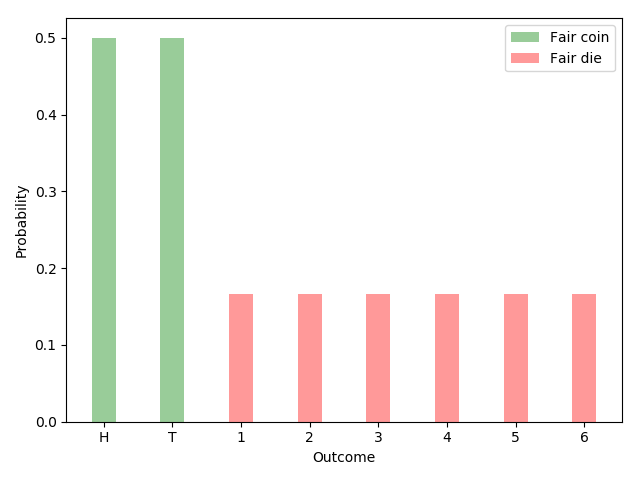Uniform distributions have maximum entropy for a given number of outcomes.

A good measure of uncertainty achieves its highest values for uniform distributions. Entropy satisfies the criterion. Given possible outcomes, maximum entropy is maximized by equiprobable outcomes:

Here is the plot of the Entropy function as applied to Bernoulli trials (events with two possible outcomes and probabilities p and 1-p):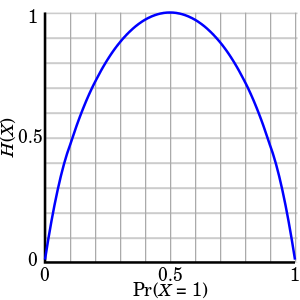In the case of Bernoulli trials, entropy reaches its maximum value for p=0.5

## Basic property 2: Uncertainty is additive for independent events

Let A and B be independent events. In other words, knowing the outcome of event A does not tell us anything about the outcome of event B.

The uncertainty associated with both events — this is another item on our wish list — should be the sum of the individual uncertainties:

Let’s use the example of flipping two coins to make this more concrete. We can either flip both coins simultaneously or first flip one coin and then flip the other one. Another way to think about this is that we can either report the outcome of the two coin flips at once or separately. The uncertainty is the same in either case.

To make this even more concrete, consider two particular coins. The first coin lands heads (H) up with an 80% probability and tails (T) up with a probability of 20%. The probabilities for the other coin are 60% and 40%. If we flip both coins simultaneously, there are four possible outcomes: HHHTTH, and TT. The corresponding probabilities are given by [ 0.48, 0.32, 0.12, 0.08 ].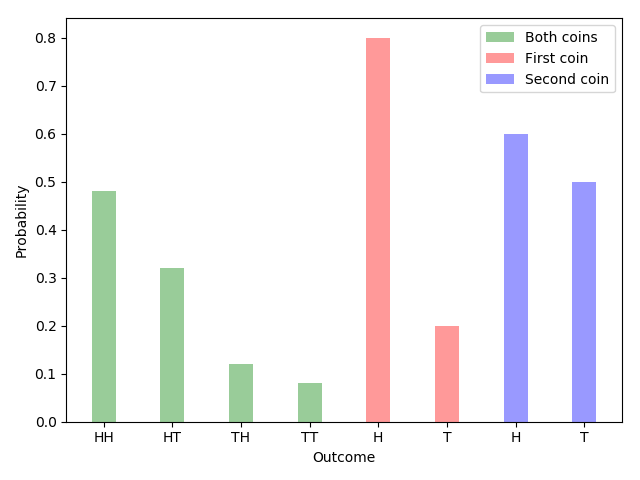The joint entropy (green) for the two independent events is equal to the sum of the individual events (red and blue).

Plugging the numbers into the entropy formula, we see that:

Just as promised.

## Basic property 3: Adding an outcome with zero probability has no effect

Suppose (a) you win whenever outcome #1 occurs and (b) you can choose between two probability distributions, A and B. Distribution A has two outcomes: say, 80% and 20%. Distribution B has three outcomes with probabilities 80%, 20%, and 0%.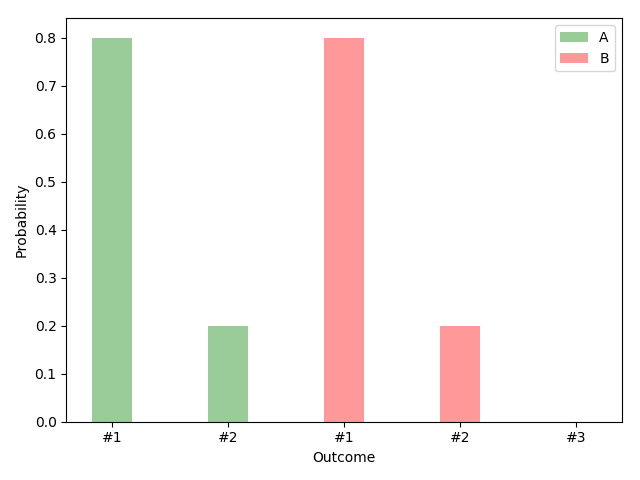Adding a third outcome with zero probability doesn’t make a difference.

Given options A and B, which one would you choose? An appropriate reaction at this point would be to shrug your shoulders or roll your eyes. The inclusion of the third outcome neither increases nor decreases the uncertainty associated with the game. A or B, who cares. It doesn’t matter.

The entropy formula agrees with this assessment: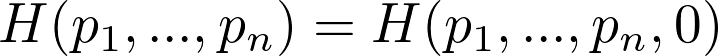Adding a zero-probability outcome has no effect on entropy.

In other words, adding an outcome with zero probability has no effect on the measurement of uncertainty.

## Basic property 4: The measure of uncertainty is continuous in all its arguments

The last of the basic properties is continuity.

Famously, the intuitive explanation of a continuous function is that there are no “gaps” or “holes”. More precisely, arbitrarily small changes in the output (uncertainty, in our case) should be achievable through sufficiently small changes in the input (probabilities).

Logarithm functions are continuous at every point for which they are defined. So are sums and products of a finite number of functions that are continuous on a subset. It follows that the entropy function is continuous in its probability arguments.

# The Uniqueness Theorem

Khinchin (1957) showed that the only family of functions satisfying the four basic properties described above is of the following form: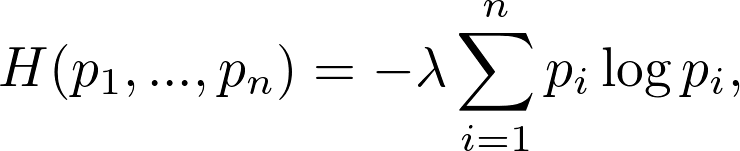Functions that satisfy the four basic properties

where λ is a positive constant. Khinchin referred to this as the Uniqueness Theorem. Setting λ = 1 and using the binary logarithm gives us the Shannon entropy.

To reiterate, entropy is used because it has desirable properties and is the natural choice among the family functions that satisfy all items on the basic wish list (properties 1–4). (I might discuss the proof in a separate article in the future.)

# Other properties

Entropy has many other properties beyond the four basic ones used in Khinchin’s Uniqueness Theorem. Let me just mention some of them here.

## Property 5: Uniform distributions with more outcomes have more uncertainty

Suppose you have the choice between a fair coin and a fair die:

And let’s say you win if the coin lands head up or the die lands on face 1.

Which of the two options would you choose? A if you are a profit maximizer and B if you prefer with more variety and uncertainty.

As the number of equiprobable outcomes increases, so should our measure of uncertainty.

And this is exactly what Entropy does: H(1/6, 1/6, 1/6, 1/6, 1/6, 1/6) > H(0.5, 0.5).

And, in general, if we let L(k) be the entropy of a uniform distribution with k possible outcomes, we have

for m > n.

## Property 6: Events have non-negative uncertainty

Do you know what negative uncertainty is? Neither do I.

A user-friendly measure of uncertainty should always return a non-negative quantity, no matter what the input is.

This is yet another criterion that is satisfied by entropy. Let’s take another look at the formula:

Probabilities are, by definition, in the range between 0 and 1 and, therefore, non-negative. The logarithm of a probability is non-positive. Multiplying the logarithm of a probability with a probability doesn’t change the sign. The sum of non-positive products is non-positive. And finally, the negative of a non-positive value is non-negative. Entropy is, thus, non-negative for every possible input.

## Property 7: Events with a certain outcome have zero uncertainty

Suppose you are in possession of a magical coin. No matter how you flip the coin, it always lands head up.

How would you quantify the uncertainty about the magical or any other situation in which one outcome is certain to occur? Well, there is none. So the natural answer— I think, you will agree — is 0.

Does entropy agree with this intuition? Of course.

Suppose that outcome i certain is certain to occur. It follows that p_i, the probability of outcome i, is equal to 1. H(X), thus, simplifies to: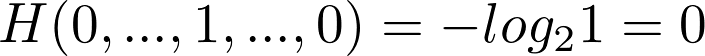The entropy for events with a certain outcome is zero.

## Property 8: Flipping the arguments has no effect

This is another obviously desirable property. Consider two cases. In the first case, the probability of heads and tails are 80% and 20%. In the second case, the probabilities are reversed: heads 20%, tails 80%.

Both coin flips are equally uncertain and have the same entropy: H(0.8, 0.2) = H(0.2, 0.8).

In more general terms, for the case of two outcomes, we have:

This fact applies to any number of outcomes. We can position the arguments (i.e., the probabilities of a distribution) in any order we like. The result of the entropy function is always the same.

Entropy and Information Gain are super important in many areas of machine learning, in particular, in the training of Decision Trees.

In his 1948 paper “A Mathematical Theory of Communication”, Claude Shannon introduced the revolutionary notion of Information Entropy.

# Entropy in Physics

Entropy, so far, had been a concept in physics. Namely, it is the (log of the) number of microstates or microscopic configurations. In colloquial terms, if the particles inside a system have many possible positions to move around, then the system has high entropy, and if they have to stay rigid, then the system has low entropy.

For example, water in its three states, solid, liquid, and gas, has different entropies. The molecules in ice have to stay in a lattice, as it is a rigid system, so the ice has low entropy. The molecules in water have more positions to move around, so water in a liquid state has medium entropy. The molecules inside water vapor can pretty much go anywhere they want, so water vapor has high entropy.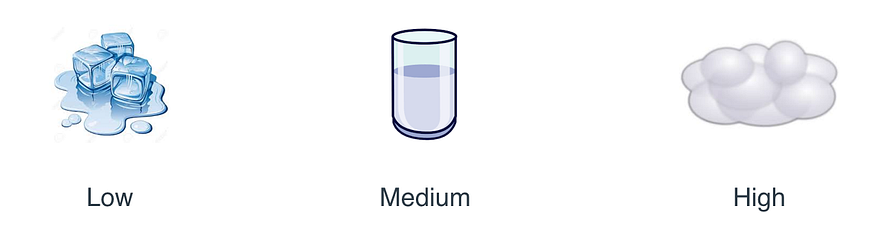Water in its three states, and their respective entropies

But what does this have to do with information theory? Well, the answer for this is by studying the relationships between knowledge and probability.

# Entropy and Knowledge

To introduce the notion of entropy in probability, we’ll use an example throughout this whole article. Let’s say we have 3 buckets with 4 balls each. The balls have the following colors:

1. Bucket 1: 4 red balls
2. Bucket 2: 3 red balls, and 1 blue ball
3. Bucket 3: 2 red balls, and 2 blue balls

And we’ll judge these three options by how much information we have on the color of a ball drawn at random. In this case, we have the following:

1. In the first bucket, we’ll know for sure that the ball coming out is red.
2. In the second bucket, we know with 75% certainty that the ball is red, and with 25% certainty that it’s blue.
3. In the third bucket, we know with 50% certainty that the ball is red, and with the same certainty that it’s blue.

So it makes sense to say that Bucket 1 gives us the most amount of “knowledge” about what ball we’ll draw (because we know for sure it’s red), that Bucket 2 gives us some knowledge, and that Bucket 3 will give us the least amount of knowledge. Well, Entropy is in some way, the opposite of knowledge. So we’ll say that Bucket 1 has the least amount of entropy, Bucket 2 has medium entropy, and Bucket 3 has the greatest amount of entropy.

But we want a formula for entropy, so in order to find that formula, we’ll use probability.

# Entropy and Probability

So now the question is, how do we cook up a formula which gives us a low number for a bucket with 4 red balls, a high number for a bucket with 2 red and 2 blue balls, and a medium number for a bucket with 3 red and 1 blue balls? Well, as a first attempt, let’s remember the definition of entropy: If molecules have many possible rearrangements, then the system has high entropy, and if they have very few rearrangements, then the system has low entropy. So a first attempt would be to count the number of rearrangements of these balls. In this case, we have 1 possible rearrangement for Bucket 1, 4 for Bucket 2, and 6 for Bucket 3, this number is given by the binomial coefficient.Number of rearrangements for the balls in each bucket

This number of arrangements won’t be part of the formula for entropy, but it gives us an idea, that if there are many arrangements, then entropy is large, and if there are very few arrangements, then entropy is low. In the next section, we’ll cook up a formula for entropy. The idea is, to consider the probability of drawing the balls in a certain way, from each bucket.

# Entropy and an Interesting Game Show

So, in order to cook up a formula, we’ll consider the following game. The spoiler is the following: The probability of winning this game will help us get the formula for entropy.

In this game, we’re given, again, the three buckets to choose from. The rules go as follows:

1. We choose one of the three buckets.
2. We are shown the balls in the bucket, in some order. Then, the balls go back in the bucket.
3. We then pick one ball out of the bucket, at a time, record the color, and return the ball back to the bucket.
4. If the colors recorded make the same sequence than the sequence of balls that we were shown at the beginning, then we win 1,000,000 dollars. If not, then we lose.

This may sound complicated, but it’s actually very simple. Let’s say for example that we’ve picked Bucket 2, which has 3 red balls, and 1 blue ball. We’re shown the balls in the bucket in some order, so let’s say, they’re shown to us in that precise order, red, red, red, blue. Now, let’s try to draw the balls to get that sequence, red, red, red, blue. What’s the probability of this happening? Well…

1. In order for the first ball to be red, the probability is 3/4, or 0.75.
2. For the second ball to be red, the probability is again 3/4. (Remember that we put the first ball back in the bucket after looking at its color.)
3. For the third ball to be red, the probability is again 3/4.
4. For the fourth ball to be blue, the probability is now 1/4, or 0.25.

As these are independent events, then the probability of the 4 of them to happen, is (3/4)*(3/4)*(3/4)*(1/4) = 27/256, or 0.105. This is not very likely. In the figures below, we can see the probabilities of winning if we pick each of the three buckets.

For the exposition, the following three figures show the probabilities of winning with each of the buckets. For Bucket 1, the probability is 1, for Bucket 2, the probability is 0.105, and for Bucket 3, the probability is 0.0625.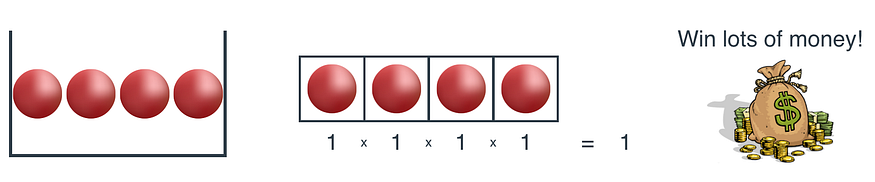The probability of winning with Bucket 1 is 1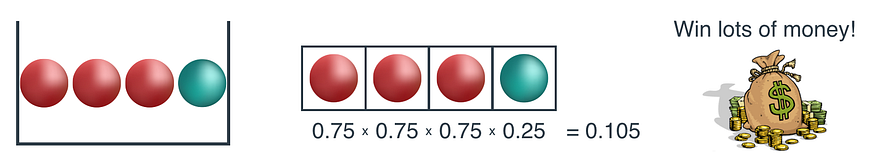The probability of winning with Bucket 2 is 0.105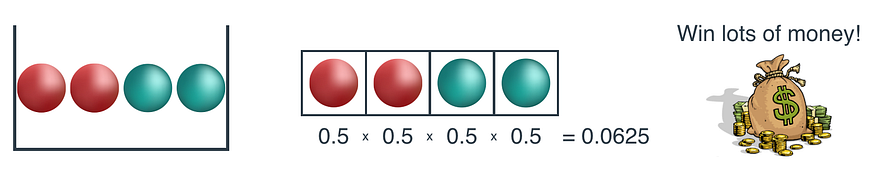The probability of winning with Bucket 3 is 0.0625

Or, as summarized in the following table:

Ok, now we have some measure that gives us different values for the three Buckets. The probability of winning this game, gives us:

1. 1.0 for Bucket 1
2. 0.105 for Bucket 2
3. 0.0625 for Bucket 3

In order to build the entropy formula, we want the opposite, some measure that gives us a low number for Bucket 1, a medium number for Bucket 2, and a high number for Bucket 3. No problem, this is where logarithms will come to save our life.

# Turning Products into Sums

The following is a very simple trick, yet used very widely, particularly in Machine Learning. See, products are never very good. Here we have a product of 4 numbers, which is not bad, but imagine if we had a million data points. How would the product of a million small probabilities (between 0 and 1) look? It would be a ridiculously tiny number. In general, we want to avoid products as much as we can. What’s better than a product? Well, a sum! And how do we turn products into sums? Exactly, using the logarithm function, since the following identity will be very helpful:

So, what do we do? Well, we have a product of four things, we take the logarithm, and that becomes the sum of four things. In the case of Bucket 2 (3 red balls, 1 blue ball), we have the following:

And taking the logarithm (in this case, we’ll take the logarithm, and multiply by -1, to make things positive), we get: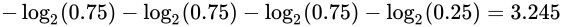For purposes of this post, we’ll take logarithm base 2, we’ll see later why (Hint: Information theory)

Now, as a final step, we take the average, in order to normalize. And that’s it, that’s the entropy! For Bucket 2, it’s 0.811:

If we calculate the entropy for Bucket 1 (4 red balls), we get:

And for Bucket 3 (2 red balls, 2 blue balls), we get:

So we have our formula for entropy, the negative logarithm of the probability of winning at our game. Notice that this is low for Bucket 1, high for Bucket 3, and medium for Bucket 2. In summary, we have the following:

For the formula lovers out there, the general formula is as follows. If our bucket has m red balls, and n blue balls, the formula is as follows:

# Multi-class Entropy

So far we’ve been dealing with two classes, red and blue. In order to relate Entropy with Information Theory, we need to look at entropy with several classes. Let’s switch to letters, to make this clear. We have the following three buckets, with 8 letters each. Bucket 1 has the letters AAAAAAAA, Bucket 2 has the letters AAAABBCD, and Bucket 3 has the letters AABBCCDD. While it’s straightforward to see that Bucket 1 has the least amount of entropy, the difference between Bucket 2 and Bucket 3 is not obvious. We’ll see below that Bucket 3 has the highest entropy of the three, while Bucket 2 has medium

The formula for entropy generalizes very easily to more classes. This is the general formula:

Where there are n classes, and p_i is the probability of an object from the i-th class appearing. For our three buckets, we have the following:

In this case, since Bucket 1 has only one class (the letter A), and the probability of it appearing is 1, then the entropy is:

For Bucket 2, since we have 4 classes (the letters A, B, C, and D), and the probability of A appearing is 4/8, for B it’s 2/8, for C it’s 1/8, and for D it’s 1/8, then the entropy is:

And finally for Bucket 3, since we have 4 classes (the letters A, B, C, and D), and the probability of each appearing is 1/4, then the entropy is:

Ok, so we’ve calculated the entropy for our three buckets.

But something much more interesting is happening, which is where information theory finally comes into play.

# Information Theory

Here’s another way to see entropy. Let’s say we want to draw a random letter from one of the buckets. On average, how many questions do we need to ask to find out what letter it is?

First, let’s get the easy case out of the way. If the bucket is Bucket 1, we know for sure that the letter is an A. So right there, we know that for Bucket 1, we need to ask 0 questions on average, to guess what letter we got. For the sake of redundancy, let’s put it in a formula: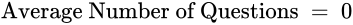Average number of questions to find out the letter drawn out of Bucket 1

Now, for buckets 2 and 3, naively, one would think that 4 questions are enough to find out any letter. Namely, the following four questions would be enough:

1. Is the letter an A?
2. Is the letter a B?
3. Is the letter a C?
4. Is the letter a D?

So, first off, the fourth question is redundant, since if the answer to all the previous ones is “no”, then we know for sure that the letter is a D. So three questions are enough. Now, can we do better than that? Well, our questions don’t need to be independent. We can tailor our question 2 based on the answer to question 1, as follows:

1. Is the letter A or B?
2. a) If the answer to question 1 is “yes”: Is the letter A? If the answer to question 1 is “no”: Is the letter C?

And that will actually do it because based on the two answers, we get the following:

1. “Yes” and “Yes”: Letter is A
2. “Yes” and “No”: Letter is B
3. “No” and “Yes”: Letter is C
4. “No” and “No”: Letter is D

This tree of questions can be seen in the following image:

Now, for Bucket 3, each letter appears with a probability 1/4, since there are 8 letters and 2 of each. Thus, the average number of questions to find out the letter drawn out of Bucket 2 is precisely 2, as the next formula states:Average number of questions to guess the letter drawn out of Bucket 2

Now, let’s look at Bucket 1. Of course, if we use the same question tree as we used for Bucket 2, we can see that the average number of questions is 2. But we can do a bit better. Actually, let’s use the first attempt. First ask if the letter is A, then B, then C. That’s the following tree: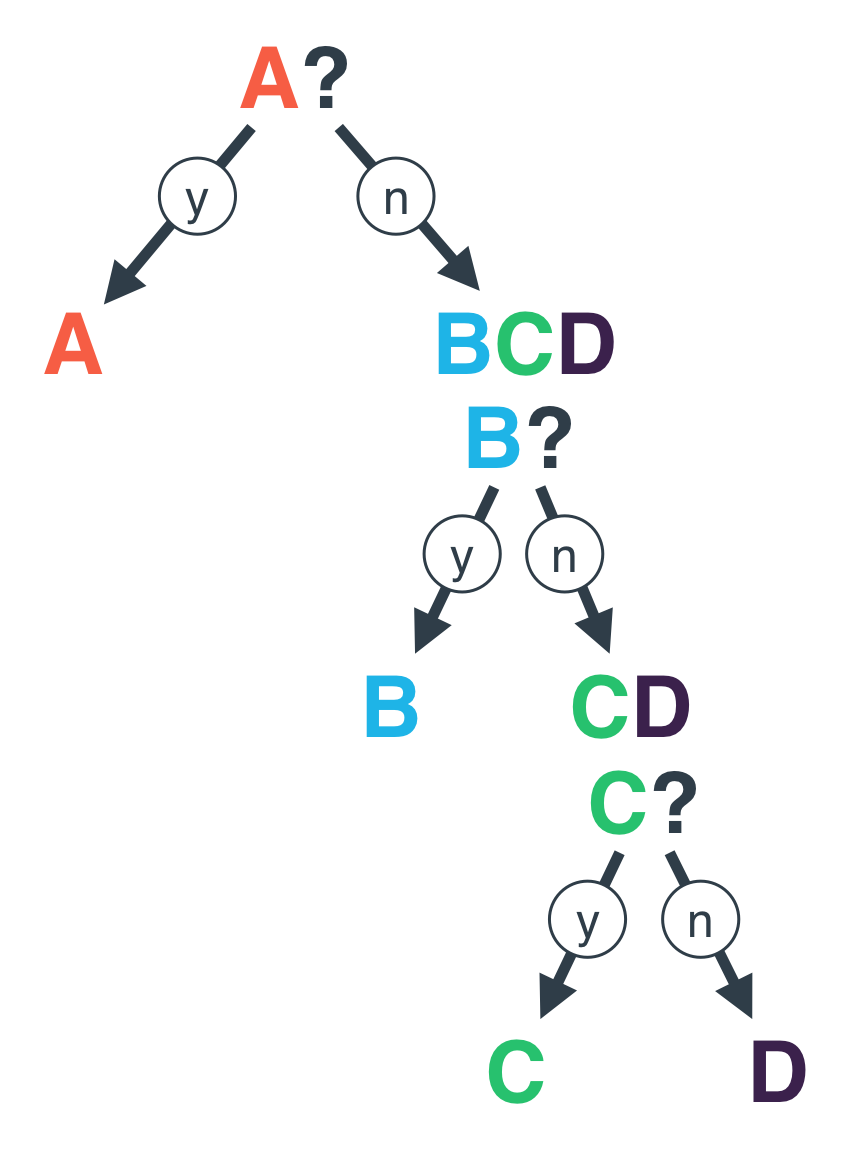Tree of questions for Bucket 2

In this case, we have the following:

1. If the letter is A, we found out in 1 question.
2. If the letter is B, we found out in 2 questions.
3. If the letter is C or D, we found out in 3 questions.

Now the trick is the following. A appears much more often than C and D, so on average, we may be doing much better. How much better? Well, recall that Bucket 2 has the letters AAAABBCD, so A appears 1/2 the time, B appears 1/4 of the time, and C and D appear each 1/8 of the time. So the average number of questions is: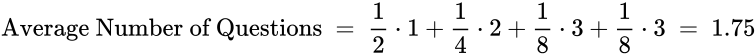Average number of questions to find out a letter drawn out of Bucket 2

So, in terms of an average number of questions asked to find out a letter drawn out of each of the buckets, we have the following:

Well, that’s exactly the entropy! Here’s the connection between Entropy and Information Theory. If we want to find out a letter drawn out of a bucket, the average number of questions we must ask to find out (if we ask our questions in the smartest possible way), is at least the entropy of the set. This means the entropy of the set is a lower bound on the number of questions we must ask in average to find out. In the cases we saw above, the number of questions is exactly the entropy. In general, this won’t happen, we may need to ask more questions than the entropy. But we will never be able to do it with fewer questions than the entropy of the set.

Of course, one huge question arises: How did we know that the way we asked the questions was the best possible? This is not obvious and needs some thinking.

# Summary

To recap, Shannon entropy is a measure of uncertainty.

It is widely used because it satisfies certain criteria (and because life is full of uncertainty). The Uniqueness Theorem tells us that only one family of functions has all the four basic properties we’ve mentioned. Shannon entropy is the natural choice among this family.

In addition to other facts, entropy is maximal for uniform distributions (property #1), additive for independent events (#2), increasing in the number of outcomes with non-zero probabilities (#3 and #5), continuous (#4), non-negative (#6), zero for certain outcomes (#7) and permutation-invariant (#8).

https://medium.com/udacity/shannon-entropy-information-gain-and-picking-balls-from-buckets-5810d35d54b4

https://towardsdatascience.com/entropy-is-a-measure-of-uncertainty-e2c000301c2c

https://machinelearningmastery.com/what-is-information-entropy/##### Amir Masoud Sefidian
Machine Learning Engineer